﻿ Static DIA Dynamic Math reflected through Prism of the Dialectic Interactive Approach

## Static DIA Dynamic Math reflected through Prism of the Dialectic Interactive Approach(Recti)linear Creative Framework for the Orientation in Time AND Space

In this creative article, it's going to be shown by this creator of knowledge that the developed concept of the simple(st) mind was DEEPLY IMPLANTED into the static (binary) math, that is, its origin is there. Or put it in another way, it might be argued, this kind of the math, interpreted in terms of its logics (logical mathematical operations), has its origin in this (basically) simplest (dynamical) concept FOR THE ORIENTATION in (recti)linear space and time. In other words, it is going to be demonstrated in a PICTORIAL way already perceived (self-)awareness by some scientists (philosophers, artists and culturologists too) that the (modern) way of thinking has been heavily influenced by the various mathematical patterns of thought ("digitalisation"), that is, the math has been deeply imprinted into the (sub)consciousness of the people. This creator of knowledge has deeper reconsidered and elaborated this basic idea by going METHODOLOGICALLY BEHIND this observed challenge in the time DIA space.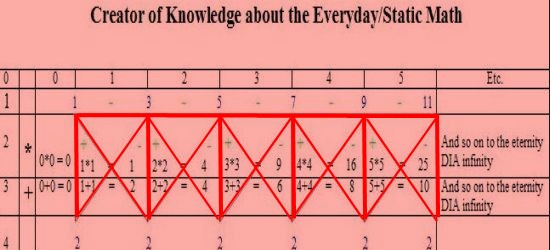In short, by using the developed powerful "tools" of the dialectic interactive approach DIA the undertaking a suitable creative traveling in time DIA space, there were deeply reconsidered the four basic operations of the dynamical mathematics (addition, multiplication, subtraction and division) from this DIALECTICAL methodological point of view. All these creative discoveries, respectively the previously mentioned mathematical (digitalised) patterns of thought, were graphically presented in the illustrations, shown in this paragraph. In other words, the developed concept of the simple(st) mind is not a naked (and fanciful) invention by this creator of knowledge, as it someone of you had thought, but rather, by being put in front of your eyes, it is become perceivable even by the five famous "scientific" senses. From this follows that the developed concept of the simple(st) mind has deep roots in the MATHEMATICAL WAY OF THINKING, understood in terms of the corresponding way of (creative) orientation in the (RECTI)LINEAR SPACE AND TIME.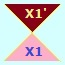Couple Dialect: Thesis DIA Antithesis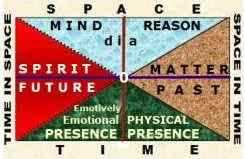Dialectical Creative Framework for Orientation in the Eternity of Times DIA Infinity of Spaces

VERSUSA Dialectical View on the Simple Mind, Seen from the Mathematical Perspective

As the first step, by reflecting it through the prism of the dialectical interactive approach, in this graphical illustration were displayed the differences (increments) of the interrelated mathematical operations of the multiplication and addition, by considering them AS A COUPLE DIALECT (thesis DIA antithesis). For example, in the row number one were displayed the increments of the mathematical operation of multiplication [shown in the row number two: 0*0=0, 1*1=1, 2*2=4, 3*3=9, etc.], as it follows: 1-0=1, 4-1=3, 9-4=5, 16-9=7, and so on. Note, if it were added (opposite operation) instead of being subtracted, the ordinal numbers of columns (cardinal numbers), which were shown in the row number zero, the result would be equal to the increments of the mathematical operation of multiplication: 0+1=1, 1+2=3, 2+3=5, 3+4 = 7, 4+5=9. After that by using this pattern of thought, in the row number four of this graphical illustration were displayed the increments of the mathematical operation of addition [see the row number three: 0+0=0, 1+1=2, 2+2=4, 3+3=6, etc.], as it follows: 2-0=2, 4-2=2, 6-4=2, 8-6=2, and so on. Finally, the increments of the mathematical operations of multiplication and addition were connected and dialectically synthesised as couple dialects into THE RIGHT-ANGLED TRIANGLES [see the row two and three]. Here it was demonstrated that the increments of each of two sides (angles) of a right-angled triangle, expressed by the related mathematical operation [successively by the subtracting (-) and addition (+)] makes the sum of the third angle. For example, the first triangle: 3-1=2, 2+1=3, 3-2=1 (thesis), the second triangle (antithesis) consists of the same factors: 3–1=2, 2+1=3, 3-2=1, making A PAIR OF THE RIGHT-ANGLED TRIANGLES [a renewed couple dialect: synthesis DIA the renewed thesis / (temporal) conclusion].

Pay your attention to the twice use of the mathematical operation of subtracting (-), whereas only once was used the mathematical operation of addition (+). You can check other triangles yourself. Note, the numbers 2 (two), in the row number four, which present a STATIC (CONSTANT) INCREMENT of the mathematical operation of addition. Pay also your attention to the coming constant (static) increment - value two (2) in the row number 1, as a prior result of the mathematical operation of multiplication, that is, 3-1=2, 5-3=2, 7-5=2, etc. In other words, THE INCREMENT OF INCREMENT of the mathematical operation of multiplication is also static (constant), that is, it is equal to the 2. Or more simply said, the mathematical operation of multiplication, after the implementation of process of the incrementing only the one step extra, was made static ("inhibited"), that is, it was deduced on the mathematical operation of addition. By the way said, if there were TRIANGULARLY connected points of these increments (for example, the increment 1 and 3) with the coming constant (static) increment of the mathematical operation of multiplication (the value 2), it would be formed an angular hollow, that is, it was revealed a hidden (lacking) triangle. In other words, in that way was completed (revealed) a degenerated "three-pointed" star (see also the graphical illustration in the case 3.4). Hope, you were able to recognise the multitude of images (sequences) of this rectilinear (creative) framework for the orientation in time and space, by transforming the dialectical contents DIA processes, into triangular forms (couples of the right-angled triangles), on this imaginable "road", formed by merging the columns 2 and 3.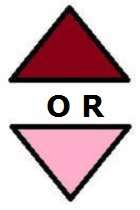Bipolar DIA Binary Way of ThinkingA Dialectical View on the Simple Mind, Seen from the Mathematical Perspective

VERSUSDialectical Creative Framework for Orientation in the Eternity of Times DIA Infinity of Spaces

In either way, such an image (couple of the right-angled triangles), that is, the sequence between any two numbers, was used as a framework for the presentation DIA interpretation of the developed concept of simple(st) mind, for example, during the process of creating knowledge. Note too, that this (creative) framework DIA the related images (right-angled triangles), is in fact a "degenerated" manifestation of the dialectic creative framework for the orientation IN TIME DIA SPACE. This dialectic creative framework was developed and presented two years earlier (1999), that is, independently of this rectilinear story, by using other sources for the inspiration, in order to be undertaken the suitable creative journey in the depth of time DIA space. By comparing these two creative frameworks, it is easily noticeable that within the rectilinear creative framework for the orientation in time and space (the rectilinear space - dimension), misses a central triangle (an empty triangular hole). In other words, by these two (creative) frameworks was actually revealed an observable difference between a DIALECTICAL TRIANGULAR APPROACH for the orientation in time DIA space versus a DYNAMICAL RIGHT-ANGLED TRIANGULAR ONE for the orientation in time and space (the rectilinear approach).

Recall, in full agreement with the dialectic interactive approach, because in its focus are the dia-processes of transition, transformation, metamorphosis and transfiguration from a state in time DIA space into an another one, everything has been presented by the (various kinds of) TRIANGLES, whereas the lines were only used as some kind of the imaginative delimiters (separators) within the initiated dia-processes. In order to be also feasible a consideration of the dynamical contents (problematic), within the developed dialectical interactive approach, there were used the right-angled triangles, as a kind of SUBSTITUTE FOR THE LINES. On the other hand, the rectilinear methodological approach, because of being based on the dual (bipolar) way of thinking, all essential processes within this imaginary line were concentrated on its two poles (0-1): The everything else between them was simply attracted by the strong "gravitational" forces of these poles, understood in terms of the mutual approaching these two different points (of views, for example) by pressing all between them (compression). In other words, all it that was not incorporated by these two (OR-OR) poles (DIA one), has been dispersed, that is, neglected, neutralised. This additionally demonstrates that the rectilinear methodological approach has truly its source of (creative) inspiration in the math (geometry): Between the two points can be drawn only one line. In short, by this imaginative line DIA its two poles was presented a typical natiocratic free(dom of) choice DIA the suitable freedom of will, seen from the rectilinear methodological perspective. But this is not in any case, the end of this endless rectilinear methodological story. In other words, this imaginative natiocratic line might be further made thicker by including the corresponding natiocratic concept of the distinguishing evil from good, and so on (that is, growing even more thicker DIA fatter), followed each TIME IN this rectilinear SPACE by the suitable regrouping the (imaginary) dots within this line, understood in terms of the previously elaborated effect of the "gravitational" forces of its two (dominated) poles (of the thinking).A Dialectical View on the Incommensurable Abyss between any Two Numbers, Seen from the Perspective of Time (X1) DIA Space (X1')EITHER in terms of the Rectilinear Time OR in terms of the Rectilinear Space

As an example, note too, although the concept of the natural numbers, or real numbers, if you so will, can be presented DIA interpreted in mathematical ("static") terms as a RECTILINEAR array, that is, either as a right-angled triangular "road" of numbers (dialectic interactive approach), or as a continual line (rectilinear approach), the dialectic interactive approach, by reflecting this dialectical content through the core of its prism, here also HAS BROUGHT ON THE LIGHT OF DAY a triangular hollow (the lacking triangle: abyss) between any two numbers on this mathematically made rectilinear dot - line, that is, these rectilinear intervals of time DIA the related distances of space between the DOTS (numbers) were transformed in triangular (incommensurable) forms (triangles). In other words, this imaginative line (array) of numbers was artificially made by connecting (compressing) all dots on it, respectively in mathematical terms by rounding [that is, by transforming dots of this imaginary line in the small "circles": The building blocks of the coming into being point-punctures (poras dia sporas) of] these imaginable distances of space (and intervals of time) between them, that in fact separates any two number's values. This shows, seen from the perspective of the eternity of times DIA infinity of spaces, that not only the circular creations (pi - value) are irrational (incommensurable) but the same (might be argued) is also valid for any (recti)linear form. Or more simply said, although it is theoretically feasible to be made static a dynamical process, understood in terms of THE TIME AND SPACE, for example in the mathematical terms, in the living reality (practice), the time DIA space cannot be ULTIMATELY stopped because of its previously mentioned DIALECTICAL FEATURES and processes (a continuous dia-process of change). On the other hand, it might be argued that the "kingdom of numbers" also mimics and reflects the expansion of time DIA space (universe).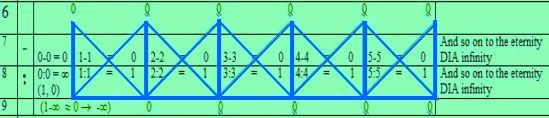Mathematical Operations Subtraction and Division

The mathematical operations subtraction and division, expressed in terms of increments (the rows number 6 and 9), are very STATIC (0), that is, do not react on the change in this static time and space. As a consequence of it, it might be argued that mathematical operations addition and especially, the multiplication initiate and drive the process of dynamisation in terms of the notion infinity (∞), whereas the task of mathematical operations division and subtracting is to stabilise these dia-processes in terms of zero (0), accordingly to do deducing them DIA the control of their "escape" velocity in the realm of "mind" (possible). By the previously elaborated was in fact interpreted and presented a triangular version (story) of the everyday (static) math, through the reinterpretation of these four (4) basic mathematical operations, based on the "linear" way of thinking, on their way from the zero to plus infinity (0 --> + 1, 2, 3, 4, .... ), forming in this way a road of triangles. Note, that this "triangular line" or more properly said the displayed endless "road" of triangles (surface) was imaginarily drawn by the rows number two and three (multiplication and addition), and, by the rows number seven and eight (subtracting and division) of this table. In other words, if I - thou - we express the deeply impressed and imprinted linear DIA dual way of thinking in my - thy -our (sub)conscious, interpreted and presented by the everyday language from zero to plus infinity (0 --> +∞), understood in terms of imaginative point-punctures between (among) numbers, I - thou - we uttered the so-called natural numbers in terms of the road of (the right-angled) triangles. Hope, it is not difficult for thou to simulate (emulate) its inverse content, that is, the story from the zero to the minus infinity (0 --> -∞) in order to be completed this story about the real numbers, respectively, to be reinterpreted it in a familiar way of thinking to me - thou - us. Or put it in another way, it was re-straightened this TRIANGULAR story about the everyday math in terms of the road of triangles that passes through zero (0), from minus infinity in direction to the plus infinity (-∞ --> 0 --> +∞), understood in terms of the myriads of imaginative point-punctures.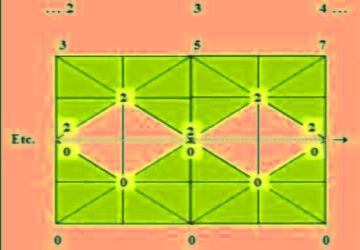Four Basic Mathematical Operations presented like a Road of Triangles

DIA

Gravitational Abysses ("0" - Black Holes) Bounded with the Numbers (Values) TWO (2) AND ZERO (0)

As a pattern of thought during your orientation in time and space, concentrate your attention on the lesser value of the increment on this imaginative road [for example, on the increment 1, comparing it to the increment 3]. In this way, it will be interpreted and presented a triangular story of this "line" (road) of triangles from zero to minus infinity (0 --> -∞), as it follows: For the mathematical operation of multiplication (0*0=0, -1*-1=1, -2*-2=4, etc.) and addition (0+0=0, -1+(-1)=-2, -2+(-2)=-4, etc.): the first triangle: 1-3=-2, +1- (-2)=3, -2+3=1, the second one, a complementary triangle, consists of the same factors: 1-3=-2, +1- (-2)=3, -2+3=1. The mathematical operations of subtraction and division, expressed in terms of the increments in the range from zero to the minus infinity (0 --> -∞), are also very static, that is, they do not reflect any change in this very static space and time too. Pay your attention that the mathematical operation of multiplication also does not reflect any change within its (negative) range, that is, its factors and increments keep positive value (negation of negation). From the all previously said, it follows that the RECTILINEAR story in the range from the zero to the minus infinity (0 --> -∞) was conveyed and preserved within this triangular story, that is, within this endless "line" (road) of the triangles, which starts from zero to the plus infinity (0 --> +∞).

READ the Next Part of this Creative Article: